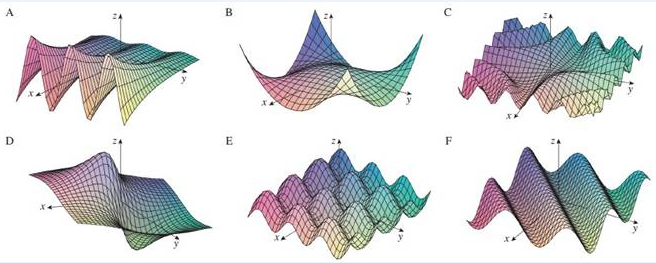Chapter 14.1, Problem 66E

Chapter
Section
Textbook Problem

Match the function (a) with its graph (labeled A−F below) and (b) with its contour map (labeled I−VI). Give reasons for your choices.66. z = x − y 1 + x 2 + y 2To determine

To match: The function z=xy1+x2+y2 .with its graph and contour map.

Explanation

Given:

The function z=xy1+x2+y2 .

Reason:

The given function z=xy1+x2+y2 is not periodic.

Also, the values of z approaches 0 as xandy approachs the maximum values

Still sussing out bartleby?

Check out a sample textbook solution.

See a sample solution

The Solution to Your Study Problems

Bartleby provides explanations to thousands of textbook problems written by our experts, many with advanced degrees!

Get Started

Solve the equations in Exercises 112 for x (mentally, if possible). 7x+55=98

Finite Mathematics and Applied Calculus (MindTap Course List)

Draw a polygon for the distribution of scores shown in the following table. X f 6 2 5 5 4 3 3 2 2 1

Essentials of Statistics for The Behavioral Sciences (MindTap Course List)

Evaluate the integral. 0/2cos2d

Calculus (MindTap Course List)

True or False: The vector function is continuous at t = 3.

Study Guide for Stewart's Multivariable Calculus, 8th

True or False: limx6x3+8x29x+75x34x+30=limx6x35x3.

Study Guide for Stewart's Single Variable Calculus: Early Transcendentals, 8th